2018-12-02 10:55:25 shanwenkang 阅读数 1424
• ###### Java经典算法讲解

在面试中，算法题目是必须的，通过算法能够看出一个程序员的编程思维，考察对复杂问题的设计与分析能力，对问题的严谨性都能够体现出来。一个算法的好坏，直接影响一个方法调用的性能，进而影响软件的整体性能。算法是学习所有编程语言的基础，在Java的学习过程中首先也会选择以算法起步，本次课程重点讲解Java开发中常用的基本算法。

29961 人正在学习 去看看 张中强

# 阈值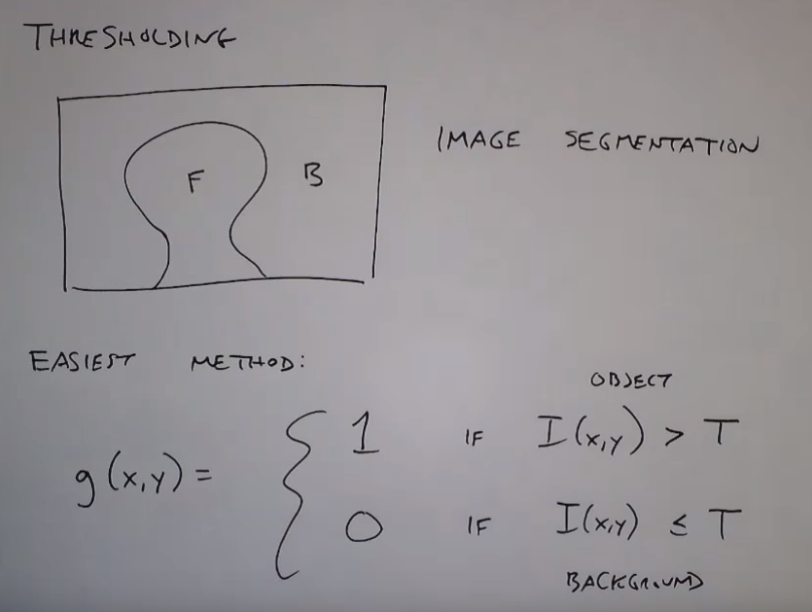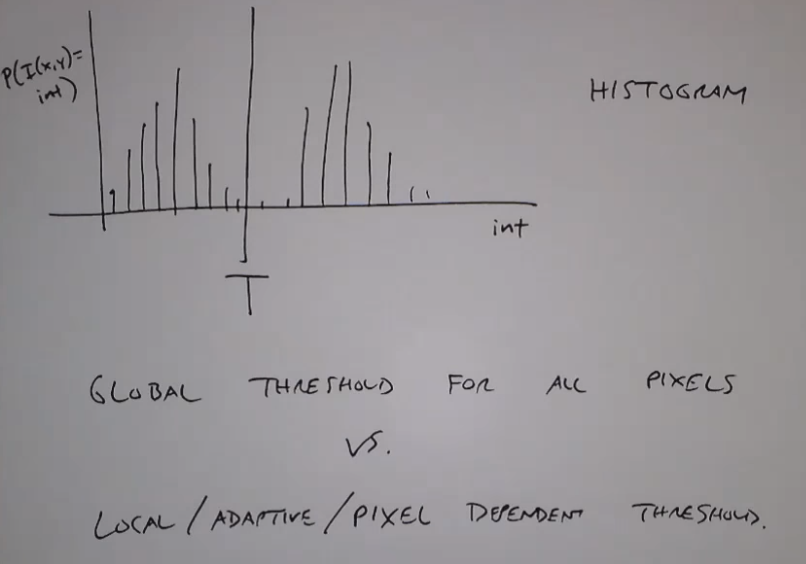# 全局阈值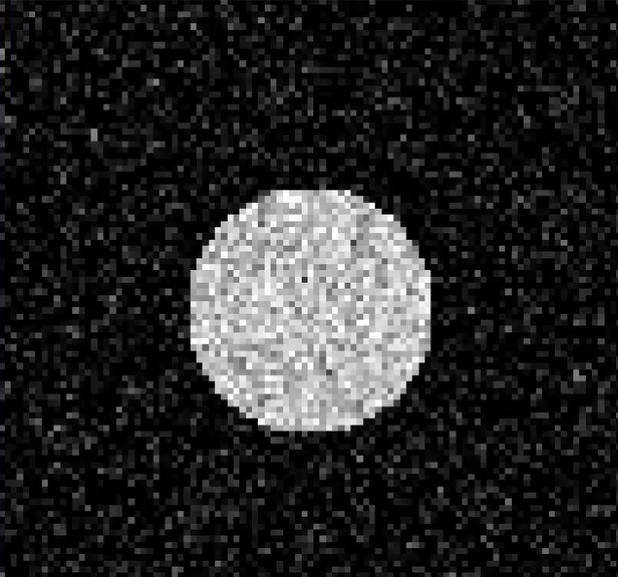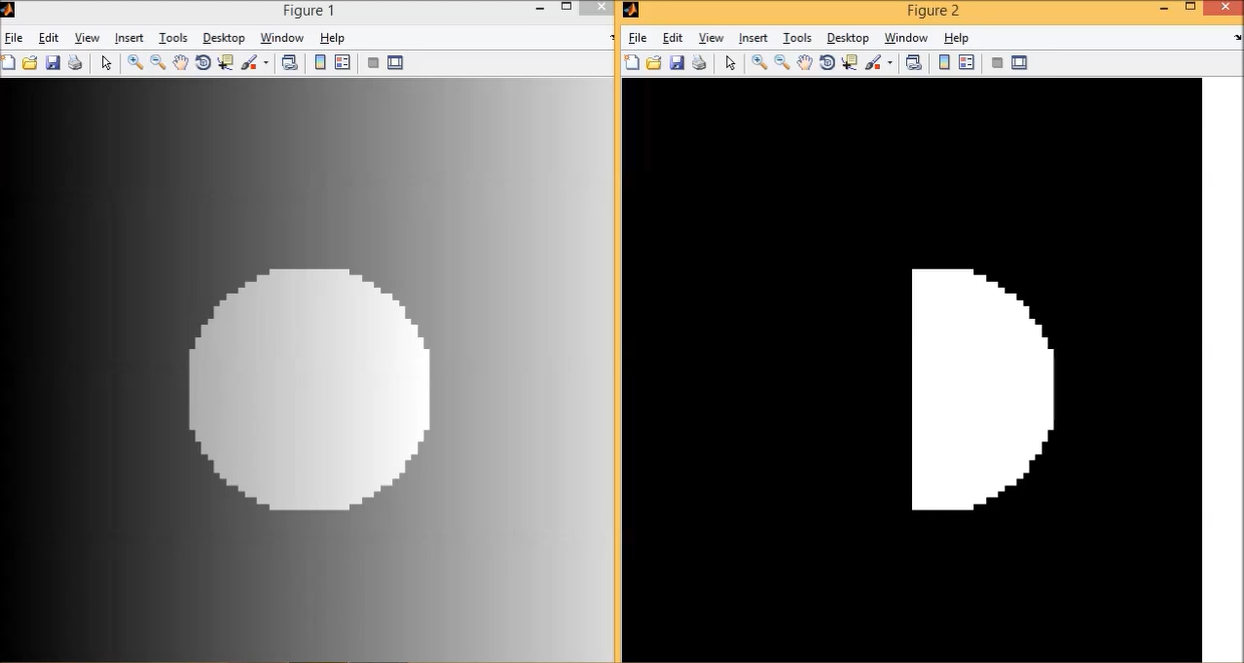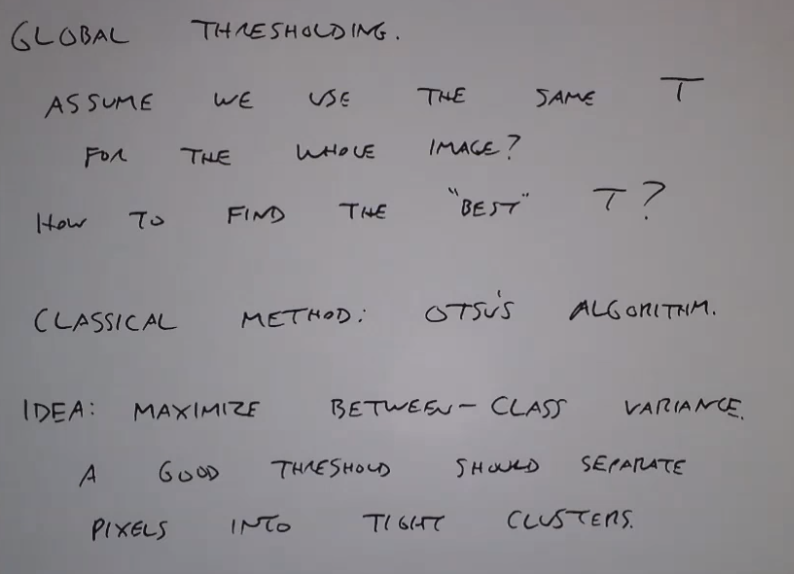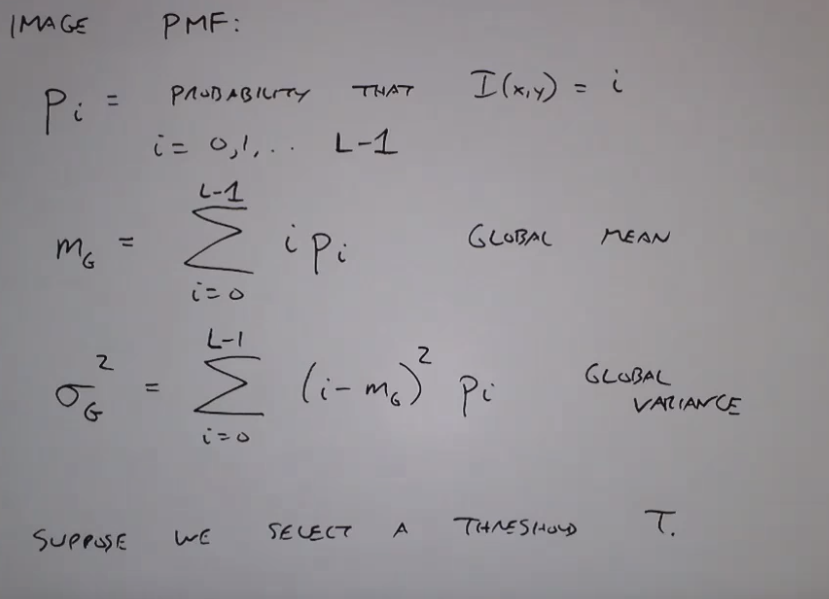OTSU算法就是想把图像分为两个块，然后使得这两个块之间的方差最大，也就是最大化两个块的均值与全局均值的差的平方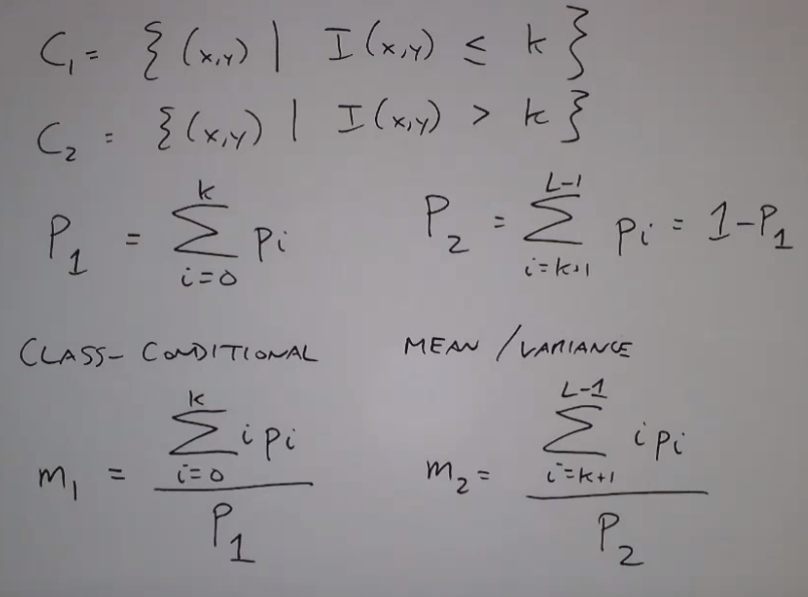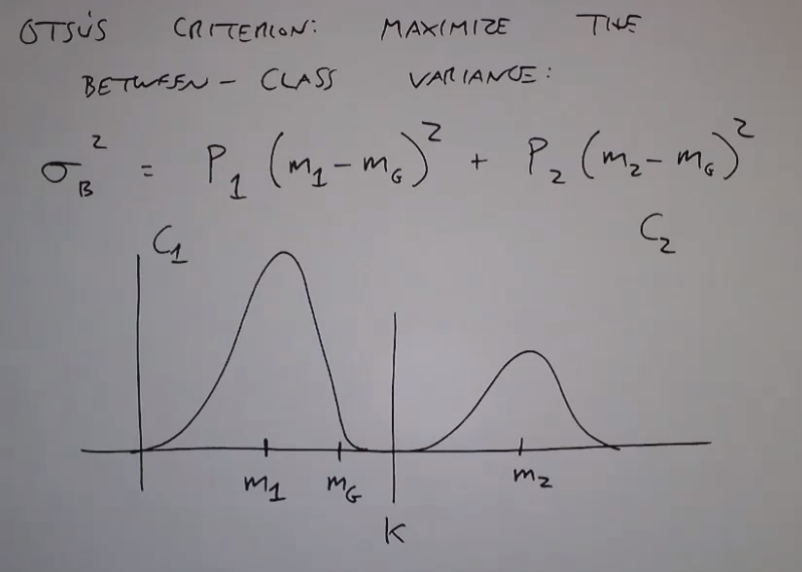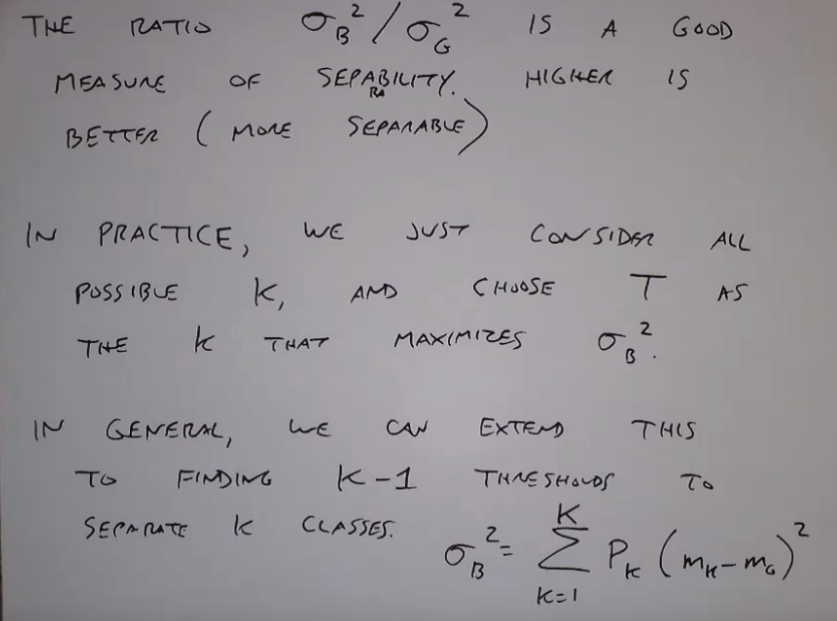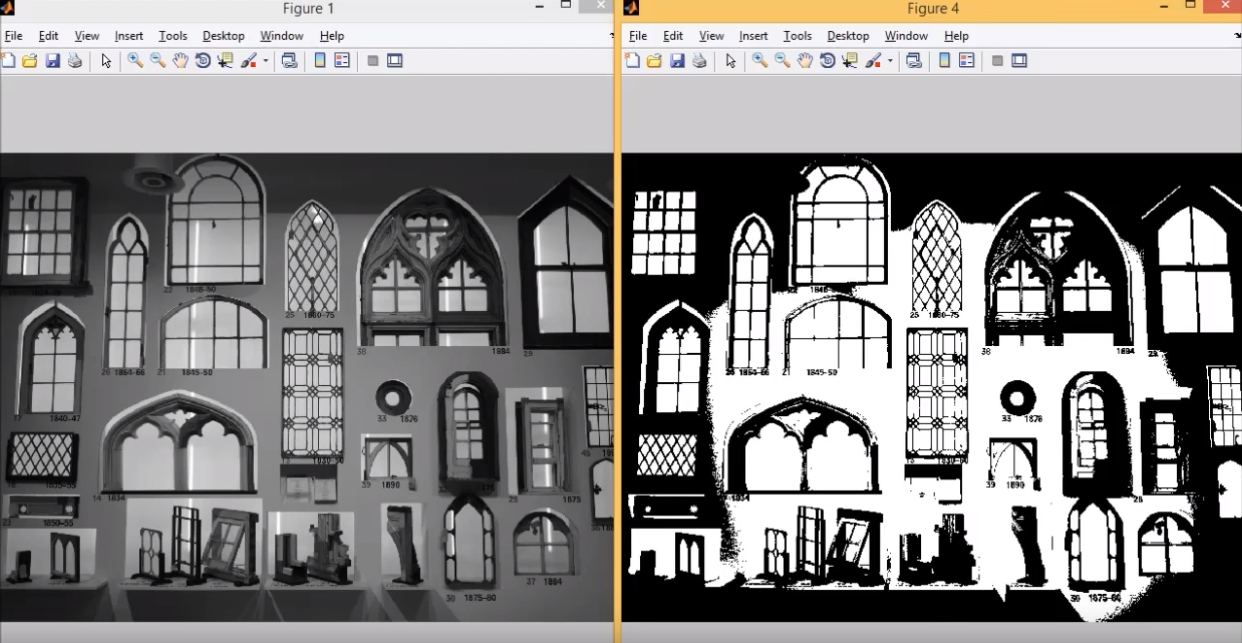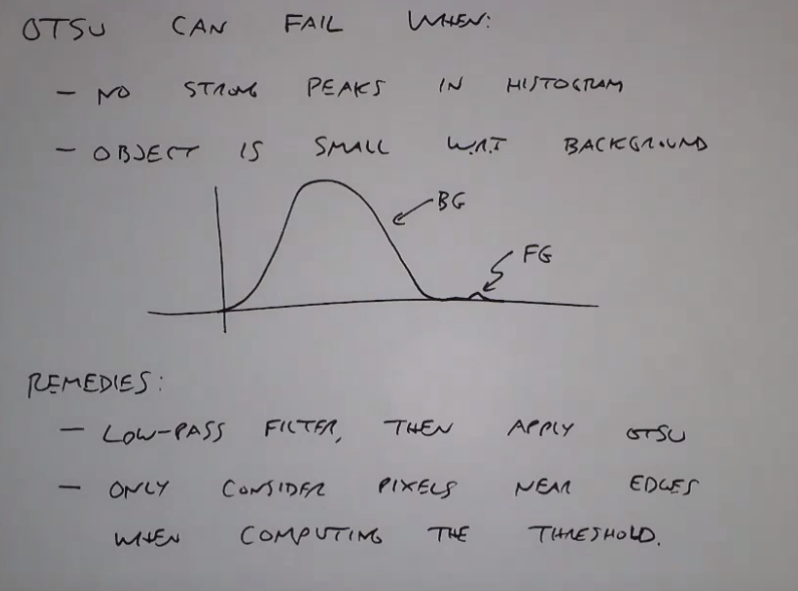## 局部阈值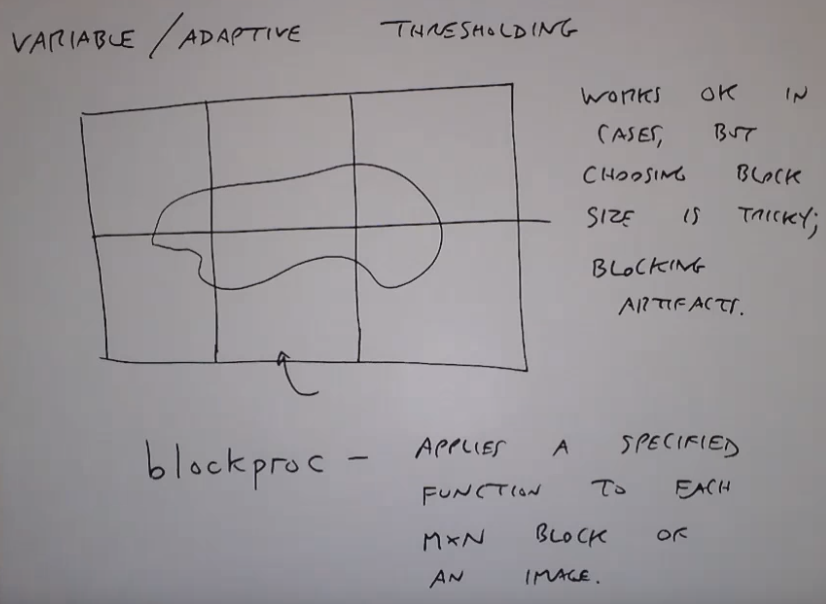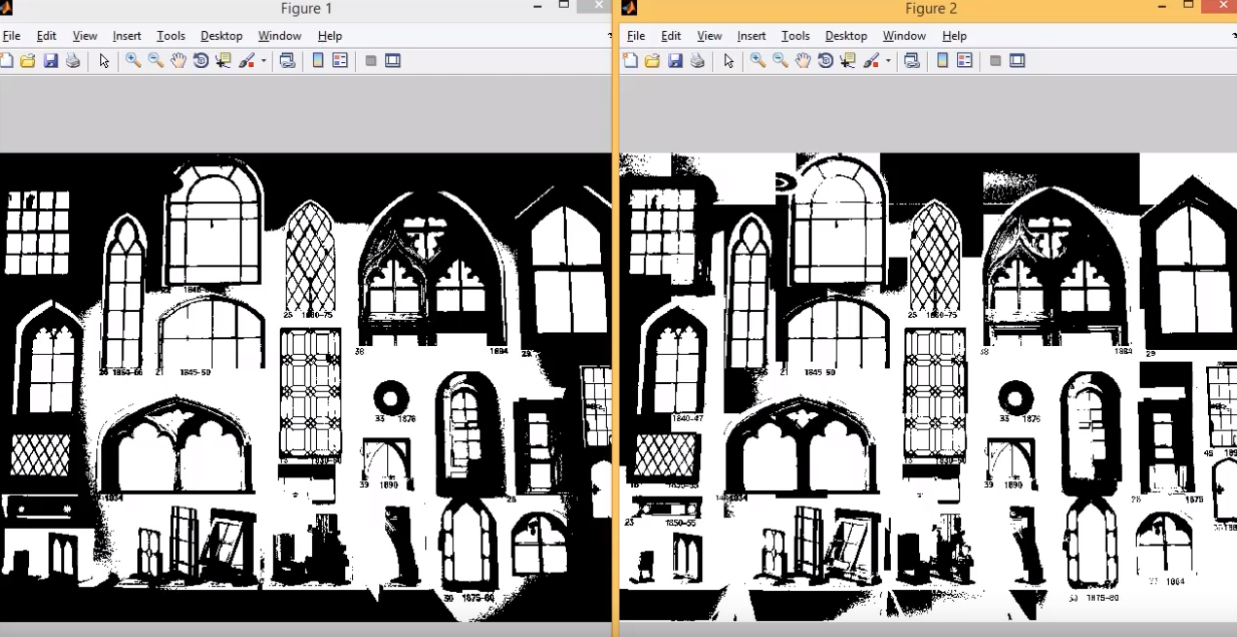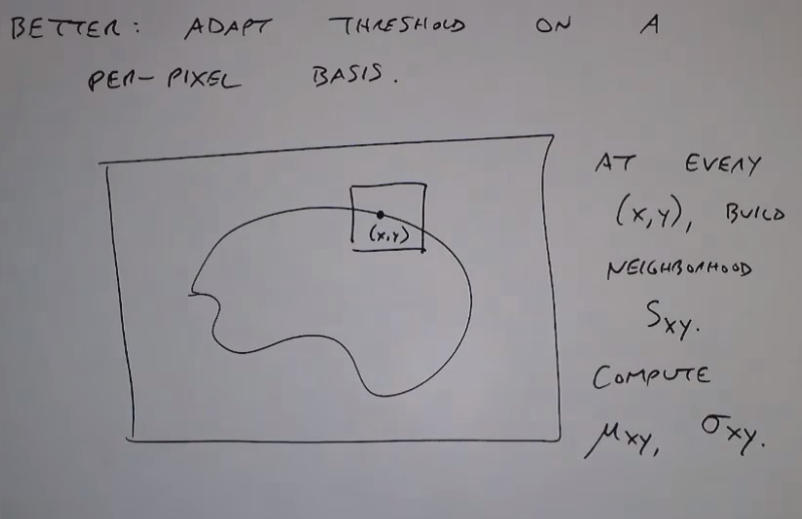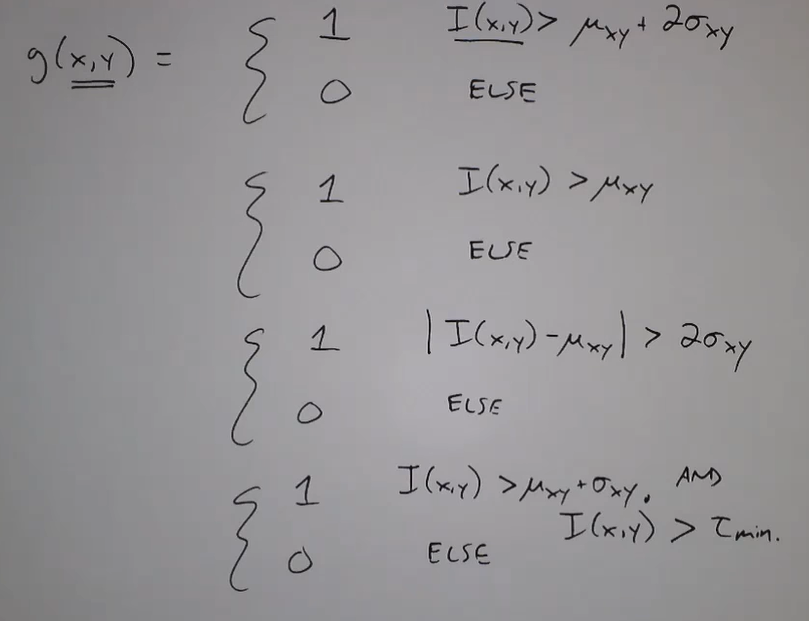### RGB图阈值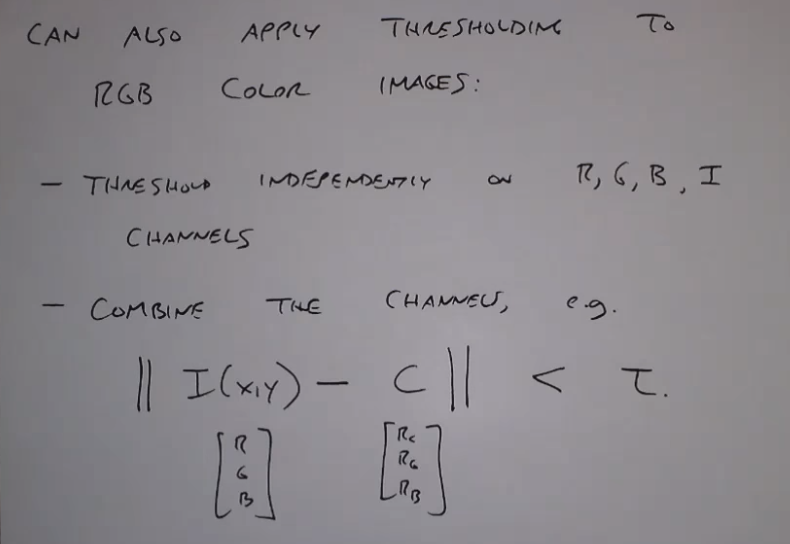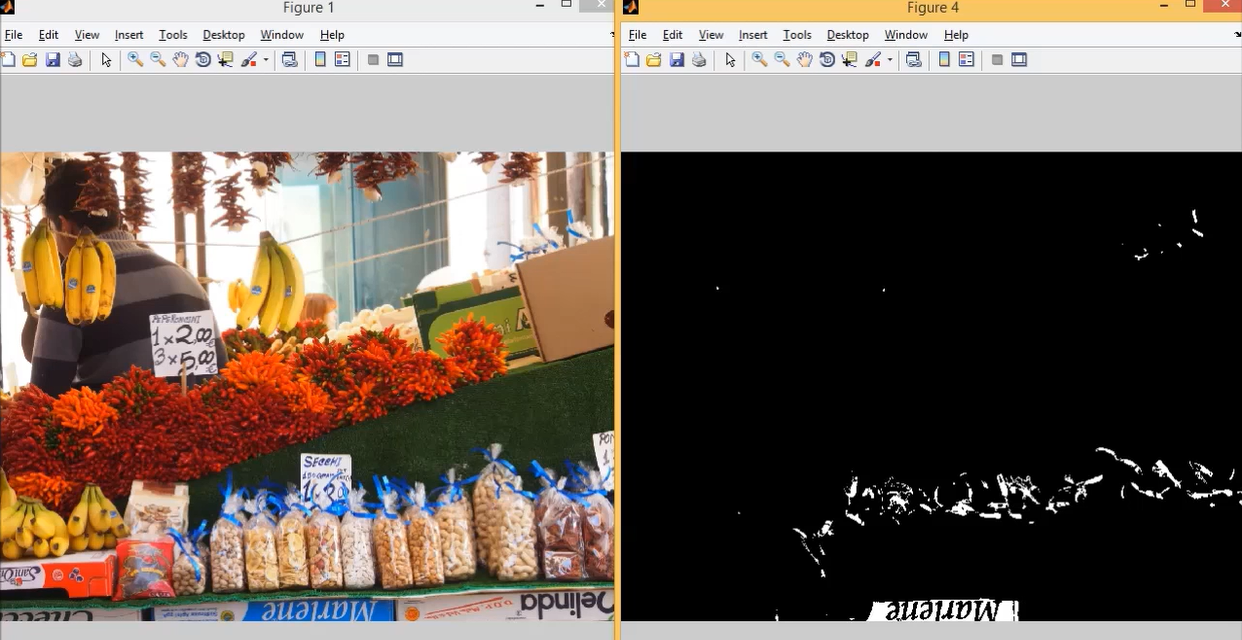2013-03-13 13:10:48 skywen123456789 阅读数 93
• ###### Java经典算法讲解

在面试中，算法题目是必须的，通过算法能够看出一个程序员的编程思维，考察对复杂问题的设计与分析能力，对问题的严谨性都能够体现出来。一个算法的好坏，直接影响一个方法调用的性能，进而影响软件的整体性能。算法是学习所有编程语言的基础，在Java的学习过程中首先也会选择以算法起步，本次课程重点讲解Java开发中常用的基本算法。

29961 人正在学习 去看看 张中强

1.数字图像分割方法概要
2.基于边界分割

2.1边缘检测

2.2边界提取（简单连接，启发式搜索，曲线拟合）

3.基于区域分割

3.1阀值分割（直方图双峰，迭代法，Ostu(大律）法，基于熵的二值方法）

3.2区域生长

3.3区域分裂与合并
4.总结与实验实现（Java语言）

§1 数字图像分割处理根据不同特征，分为两类：基于边界分割和基于区域分割，主要方法有：

§2 基于边界分割

§2.1 边缘检测§2.2 边界获取

|e(xi,yi)－e(xj,yj)|<T1

|ф(xi,yi)－ф(xj,yj)|mod 2π<T2

|e(xi,yi)|>T, |e(xj,yj)|>THough 变换§3.1 阈值化分割方法

§3.1.1直方图阈值的双峰法灰度级 i出现的概率为：§3.1.2迭代法（最佳阀值分割迭代法）

(1)选取一个初始阈值T。

(2)利用阈值T把给定图像分割成两组图像，记为和。

(3)计算和均值和。

(4)选取新的阈值T,且(5)重复第(2)~(4)步，直至和均值和不再变化为止。

§3.1.3大律法(Otsu阀值分割算法）§3.1.4 类内方差最小方差法§3.1.5 最小错误概率分类法是前景与背景点数量的比例，如果方差相同，比例也相同§3.1.6 基于熵的二值化方法

O区：B区：对图像每个灰度级分别计算§3.2 基于区域的图像分割

§3.2.1 区域生长和区域合并

1. 用某种方法把图像分割成许多小区域。

2. 定义合并相邻区域的准则。

3. 按照合并准则合并所有相邻的区域，如果没有再能够合并的块后停止。

(1) 灰度差准则

①对图像进行逐步扫描，找出尚没有归属的像素；

②以该像素为中心检查它的邻域像素，将邻域中的像素逐个与它比较，如果灰度差小于预先确定的值，将它们合并；

③以新合并的像素为中心，返回到步骤②，检查新像素的邻域，直到区域能进一步扩张；

④返回到步骤①，继续扫描直到不能发现没有归属的像素，则结束整个生长过程。

①设灰度差的阈值为零，用上述方法进行区域扩张，使灰度相同像素合并；

②求出所有邻接区域之间的平均灰度差，并合并具有最小灰度差的邻接区域；

③设定终止准则，通过反复进行上述步骤②中的操作将区域依次合并直到终止准则满足为止。1)设区域为均匀的，各像素灰度值为m与一个零均值高斯噪音的叠加。当测试某个像素是否合并时，条件不成立的概率为:2)设区域为非均匀，且由两部分不同目标的图像像素构成。这两部分像素在R中所占比例分别为,灰度值分别为，则区域均值为。对灰度值为m的像素，它与区域均值的差为：(2) 灰度分布统计准则

①把图像分成互不重叠的小区域；

②比较邻接区域的累积灰度直方图根据灰度分布的相似性进行区域合并；

③设定终止准则，通过反复进行步骤②中的操作将各个区域依次合并直到终止准则满足。

Kolmogorov-Smirnov检测:Smoothed-Difference检测:(3) 区域形状准则

①把图像分割成灰度固定的区域，设两邻区域的周长分别为p1和p2 ，把两区域共同边界线两侧灰度差小于给定值的那部分长度设为L，如果(t1,为预定阈值):②把图像分割成灰度固定的区域，设两邻接区域的共同边界长度为B，把两区域共同边界线两侧灰度差小于给定值的那部分长度设为L，如果(为预定阈值）§3.2.2 区域分裂§3.2.3 分裂和合并法

1. 合并具有一致属性的共根四个节点块。

2. 分开不满足一致性要求的块，上一步没有合并得块，如果它的四个子块不满足一致性，则将其分解成4 个子块。分出的子块如不满足一致性，还可以继续分解。

3. 对相邻的具有一致属性的块合并，即使不在同一层或者没有共同的父节点。将四叉树变为邻接图表示。

4. 如果没有进一步的合并或分解，算法终止。

§3.4 边缘与区域相结合的分割

1. 把所有像素点放到待处理点集合A 中

2. 如果 A 空则结束。否则从A 中任意移出一点,作为连通域a（用集合表示）的初始点。

3. 在 A 中寻找所有与a 连通的点，并移到a 中。如果没有找到，转到2，寻找下一个连通域

4. 转到 3，迭代寻找新的连同点。算法逻辑简单，速度慢。快速算法：每点只需要遍历一次。

图像分割的评价

Haralick 和Shapiro 建立了下列定性的指导：

§4.1图像分割总结

1. 图像分割是图像描述的重要基础。

2. 怎样获得分割呢？

3. 基于边界或区域的方法都是基于基本假设，假设不成立时就会遇到困难。基于边界的方法，物体内部边缘，光照等原因物体间边缘差别小或平滑过渡。人可以在理解的基础上判别，需要更上层知识的指导。基于区域的方法，区域内部特征不一定那么均匀。

4. 虽然图像处理一开始就研究分割的问题，也取得了相当进展，但尚无一种适合于所有图像的通用分割算法。

5. 人们至今仍然在努力发展新的、更有潜力的方法，期待更通用、更完美的分割结果。

6. 本章介绍了一些典型的基本的分割算法，实际上，现有的方法通常是针对具体问题。为了得到好的性能，要根据实际问题选择或设计算法。众多方法的关键是模型和条件，不同的模型和条件决定了不同的方法。根据具体任务找到或设计匹配的模型，根据条件求解。

7. 在研究新方法的同时，分割参数的自动选择，利用统计理论作决策，适用于快速处理的数据结构，较为通用的分割结果质量评价标准都是研究者关心的内容。如果区域内部灰度有剧烈的变化，则需要用纹理分析来分割。

§4.2 程序实现

1. //一维最大熵分割算法
2.     public int segment(int[] pix, int w, int h)
3.     {
4.         int i, j, t;
5.         double a1, a2, max, pt;
6.         double[] p = new double;
7.         double[] num = new double;
8.
9.         int[][] im = new int[w][h];
10.
11.         for(j = 0; j < h; j++)
12.             for(i = 0; i < w; i++)
13.                 im[i][j] = pix[i+j*w]&0xff;
14.
15.         for (i = 0; i < 256; i++)
16.             p[i] = 0;
17.
18.         //统计各灰度级出现的次数
19.         for (j = 0; j < h; j++)
20.             for (i = 0; i < w; i++)
21.                 p[im[i][j]]++;
22.
23.         /**
24.          *关于计算各灰度级出现的概率
25.          *1.因为(p[j]/(w*h)) / (pt/(w*h)) = p[j] / pt
26.          *  所以计算p[j] / pt不必计算概率
27.          *2.当p[j]=0时，计算Math.log(p[j] / pt)将出现无穷大.但
28.          *  此时p[j] / pt) * Math.log(p[j] / pt)=0
29.          *  所以在计算a1时,不必计算这一项
30.          */
31.         int hw =  h*w;
32.         for (i = 0; i < 256; i++)
33.         {
34.             a1 = a2 = 0.0;
35.             pt = 0.0;
36.             for (j = 0; j <= i; j++)
37.                 pt += p[j];
38.
39.             for (j = 0; j <= i; j++)
40.
41.                 if(p[j]>0)
42.                     a1 += (p[j]/pt) * Math.log(pt/p[j]);
43.
44.             for (j = i+1; j <256; j++)
45.                 if(p[j]>0)
46.                     a2 += (p[j] /(hw-pt))* Math.log((hw - pt)/p[j]);
47.
48.             num[i] = a1 + a2;
49.         }
50.
51.         max = 0.0; t = 0;
52.         for (i = 0; i < 256; i++)
53.         {
54.             if (max < num[i])
55.             {
56.                 max = num[i];
57.                 t = i;
58.             }
59.         }
60.         return t;
61.     }

1.   public int segment2(int[] pix, int w, int h)
2. {
3.     int i, j, u, v, t;
4.        double a1, a2, max, pa, pb, pa2, pb2, sum;
5.        double[][] p = new double;
6.        double[][] num = new double;
7.
8.     int[][] im = new int[w][h];
9.
10.     for(j = 0; j < h; j++)
11.         for(i = 0; i < w; i++)
12.             im[i][j] = pix[i+j*w]&0xff;
13.
14.        for(i = 0; i < 256; i++)
15.            for(j = 0; j < 256; j++)
16.                p[i][j] = 0;
17.
18.        //统计2维直方图p[i][j]
19.        for(j = 1; j < h-1; j++)
20.        {
21.            for(i = 1; i < w-1; i++)
22.            {
23.             t = (int)((im[i-1][j]+im[i+1][j]+im[i][j-1]
24.               +im[i][j+1]+im[i][j])/5);//4-邻域均值
25.                p[im[i][j]][t]++;
26.            }
27.        }
28.
29.        pa = 0.0; pb = 0.0; max = 0.0; t = 0;
30.        for(i = 49; i < 200; i=i+2)
31.        {
32.            System.out.println((int)(i*100/199)+" %");
33.            for(j = 0; j < 256; j++)
34.         {
35.             a1 = 0.0; a2 = 0.0;
36.             pb = 0.0;
37.
38.             //递推算法计算pa
39.             if(j != 0)
40.             {
41.                 for(u = 0; u <= i; u++)
42.                     pa += p[u][j];
43.             }
44.             else
45.             {
46.                 pa = 0.0;
47.                 for( u = 0; u <= i; u++)
48.                     pa += p[u];
49.             }
50.
51.             //递推算法计算pb
52.             if(j != 0)
53.             {
54.                 for(u = i+1;u < 256;u++)
55.                     pb -= p[u][j];
56.             }
57.             else
58.             {
59.                 pb = 0;
60.                 for(u = i+1;u < 256;u++)
61.                     for(v = j+1; v < 256; v++)
62.                         pb += p[u][v];
63.             }
64.
65.             for(u = 0; u <= i; u++)
66.                 for(v = 0; v <= j; v++)
67.                     if(p[u][v] > 0)
68.                         a1 += (double)(-p[u][v]/pa)* Math.log(p[u][v]/pa);
69.
70.             for(u = i+1; u < 256; u++)
71.                 for(v = j+1; v < 256; v++)
72.                     if(p[u][v] > 0)
73.                         a2 += (double)(-p[u][v]/pb)* Math.log(p[u][v]/pb);
74.
75.             num[i][j] = a1 + a2;
76.            }
77.        }
78.
79.        max = 0.0; t = 0;
80.        for (i = 0; i < 256; i++)
81.        {
82.         for(j = 0; j < 256; j++)
83.         {
84.                if (max < num[i][j])
85.                {
86.                    max = num[i][j];
87.                    t = i;
88.                }
89.            }
90.        }
91.        return t;
92. }

最佳阈值分割

1. public int bestThresh(int[] pix, int w, int h)
2. {
3.     int i, j, t,
4.         thresh,
5.         newthresh,
6.         gmax, gmin;         //最大,最小灰度值
7.        double a1, a2, max, pt;
8.        double[] p = new double;
9.        long[] num = new long;
10.
11.     int[][] im = new int[w][h];
12.
13.     for(j = 0; j < h; j++)
14.         for(i = 0; i < w; i++)
15.             im[i][j] = pix[i+j*w]&0xff;
16.
17.        for (i = 0; i < 256; i++)
18.            p[i] = 0;
19.
20.        //1.统计各灰度级出现的次数、灰度最大和最小值
21.        gmax = 0;
22.        gmin =255;
23.        for (j = 0; j < h; j++)
24.        {
25.            for (i = 0; i < w; i++)
26.            {
27.             int g = im[i][j];
28.                p[g]++;
29.                if(g > gmax) gmax = g;
30.                if(g < gmin) gmin = g;
31.            }
32.        }
33.
34.        thresh = 0;
35.        newthresh = (gmax+gmin)/2;
36.
37.        int meangray1,meangray2;
38.        long p1, p2, s1, s2;
39.        for(i = 0; (thresh!=newthresh)&&(i<100);i++)
40.        {
41.         thresh = newthresh;
42.         p1 = 0; p2 = 0; s1 = 0; s2 = 0;
43.
44.         //2. 求两个区域的灰度平均值
45.         for(j = gmin; j < thresh;j++)
46.         {
47.             p1 += p[j]*j;
48.             s1 += p[j];
49.         }
50.         meangray1 = (int)(p1/s1);
51.
52.         for(j = thresh+1; j < gmax; j++)
53.         {
54.             p2 += p[j]*j;
55.             s2 += p[j];
56.         }
57.         meangray2 = (int)(p2/s2);
58.         //3. 计算新阈值
59.         newthresh = (meangray1+meangray2)/2;
60.        }
61.        return newthresh;
62. }

Otsu阈值分割

1. public int otsuThresh(int[] pix, int iw, int ih)
2. {
3.     ColorModel cm = ColorModel.getRGBdefault();
4.        int wh = iw * ih;
5.        int[][] inIm = new int[iw][ih];
6.
7.        int i, j, t;
8.        int L = 256;
9.        double[] p = new double[L];
10.
11.        for (j = 0; j < ih; j++)
12.            for (i = 0; i < iw; i++)
13.                inIm[i][j] = pix[i+j*iw]&0xff;
14.
15.        for (i = 0; i < L; i++)
16.            p[i] = 0;
17.
18.        //计算各灰度出现次数
19.        for (j = 0; j < ih; j++)
20.            for (i = 0; i < iw; i++)
21.                p[inIm[i][j]]++;
22.
23.        //计算各灰度级出现概率
24.        for (int m = 0; m < L; m++)
25.            p[m] = p[m] / wh;
26.
27.        double[] sigma = new double[L];
28.        for (t = 0; t < L; t++)
29.        {
30.            double w0 = 0;
31.            for (int m = 0; m < t+1; m++)
32.                w0 += p[m];
33.            double w1 = 1 - w0;
34.
35.            double u0 = 0;
36.            for (int m = 0; m < t + 1; m++)
37.                u0 += m * p[m] / w0;
38.
39.            double u1 = 0;
40.            for (int m = t; m < L; m++)
41.                u1 += m * p[m] / w1;
42.
43.            sigma[t] = w0*w1*(u0-u1)*(u0-u1);
44.        }
45.        double max = 0.0;
46.        int T = 0;
47.        for (i = 0; i < L-1; i++)
48.        {
49.            if (max < sigma[i])
50.            {
51.                max = sigma[i];
52.                T = i;
53.            }
54.        }
55.        return T;
56. }

1. //图像序列pix阈值分割
2. public int[] thSegment(int[] pix, int iw, int ih, int th)
3. {
4.     int[] im = new int[iw*ih];
5.     int t;
6.     for(int i = 0; i < iw*ih; i++)
7.     {
8.         t = pix[i]&0xff;
9.
10.         if(t > th)
11.             im[i] = (255<<24)|(255<<16)|(255<<8)|255;//背景色
12.         else
13.             im[i] = (255<<24)|(0<<16)|(0<<8)|0;      //前景色为
14.     }
15.     return im;
16. }2018-10-30 22:19:36 Eastmount 阅读数 4994
• ###### Java经典算法讲解

在面试中，算法题目是必须的，通过算法能够看出一个程序员的编程思维，考察对复杂问题的设计与分析能力，对问题的严谨性都能够体现出来。一个算法的好坏，直接影响一个方法调用的性能，进而影响软件的整体性能。算法是学习所有编程语言的基础，在Java的学习过程中首先也会选择以算法起步，本次课程重点讲解Java开发中常用的基本算法。

29961 人正在学习 去看看 张中强

PS：请求帮忙点个Star，哈哈，第一次使用Github，以后会分享更多代码，一起加油。

1.阈值化
2.二进制阈值化
3.反二进制阈值化
4.截断阈值化
5.反阈值化为0
6.阈值化为0

PS：文章参考自己以前系列图像处理文章及OpenCV库函数，同时部分参考网易云视频，推荐大家去学习。同时，本篇文章涉及到《计算机图形学》基础知识，请大家下来补充。

PSS：2019年1~2月作者参加了CSDN2018年博客评选，希望您能投出宝贵的一票。我是59号，Eastmount，杨秀璋。投票地址：https://bss.csdn.net/m/topic/blog_star2018/index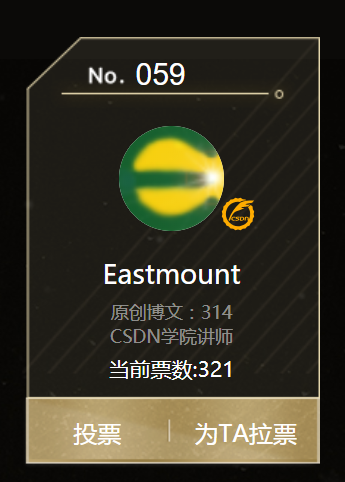# 一. 阈值化

（注：该部分参考作者的论文《基于苗族服饰的图像锐化和边缘提取技术研究》）

$\begin{cases} Y=0，gray=T\\ \end{cases}$

Python OpenCV中提供了阈值函数threshold()实现二值化处理，其公式及参数如下图所示：
retval, dst = cv2.threshold(src, thresh, maxval, type)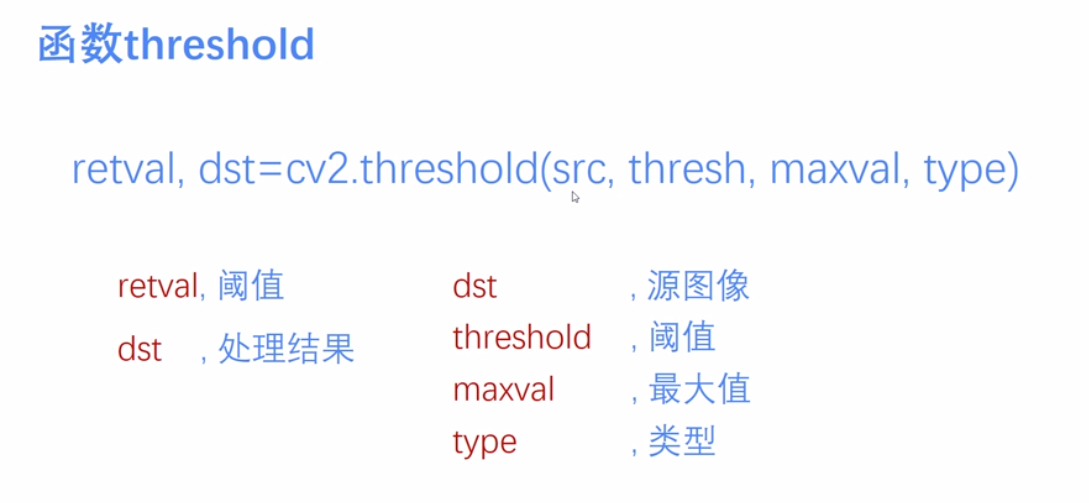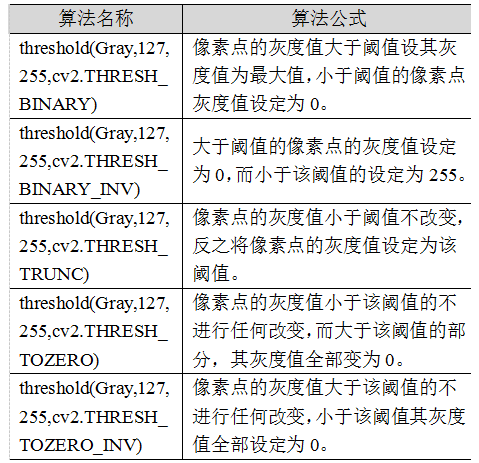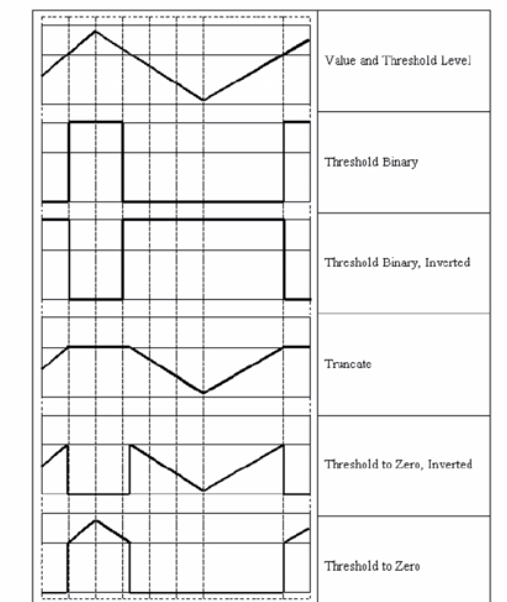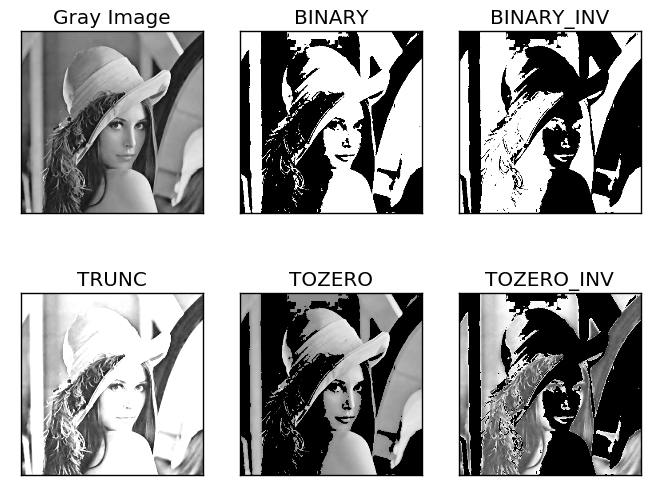# 二. 二进制阈值化

$dst(x,y) = \begin{cases} maxVal， if src(x,y)>thresh\\ 0，otherwise\\ \end{cases}$
(1) 大于等于127的像素点的灰度值设定为最大值（如8位灰度值最大为255）
(2) 灰度值小于127的像素点的灰度值设定为0

#encoding:utf-8
import cv2
import numpy as np

#读取图片

#灰度图像处理
GrayImage = cv2.cvtColor(src,cv2.COLOR_BGR2GRAY)

#二进制阈值化处理
r, b = cv2.threshold(GrayImage, 127, 255, cv2.THRESH_BINARY)
print r

#显示图像
cv2.imshow("src", src)
cv2.imshow("result", b)

#等待显示
cv2.waitKey(0)
cv2.destroyAllWindows()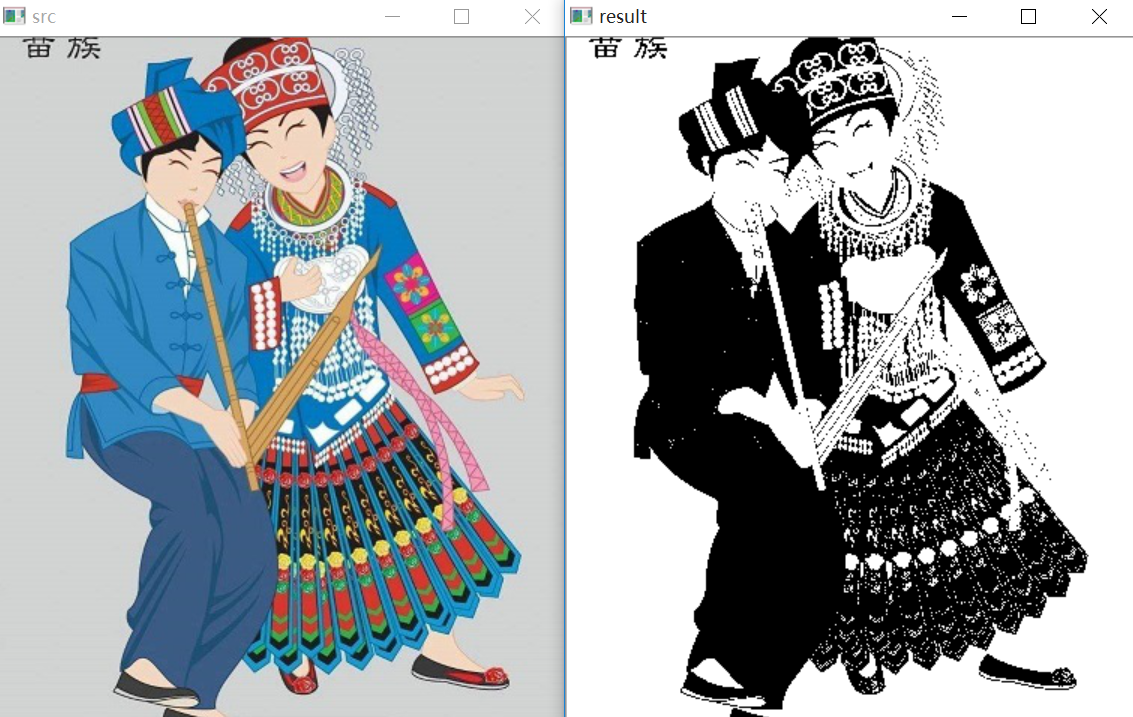# 三. 反二进制阈值化

$dst(x,y) = \begin{cases} 0， if src(x,y)>thresh\\ maxVal，otherwise\\ \end{cases}$
(1) 大于127的像素点的灰度值设定为0（以8位灰度图为例）
(2) 小于该阈值的灰度值设定为255

#encoding:utf-8
import cv2
import numpy as np

#读取图片

#灰度图像处理
GrayImage = cv2.cvtColor(src,cv2.COLOR_BGR2GRAY)

#反二进制阈值化处理
r, b = cv2.threshold(GrayImage, 127, 255, cv2.THRESH_BINARY_INV)
print r

#显示图像
cv2.imshow("src", src)
cv2.imshow("result", b)

#等待显示
cv2.waitKey(0)
cv2.destroyAllWindows()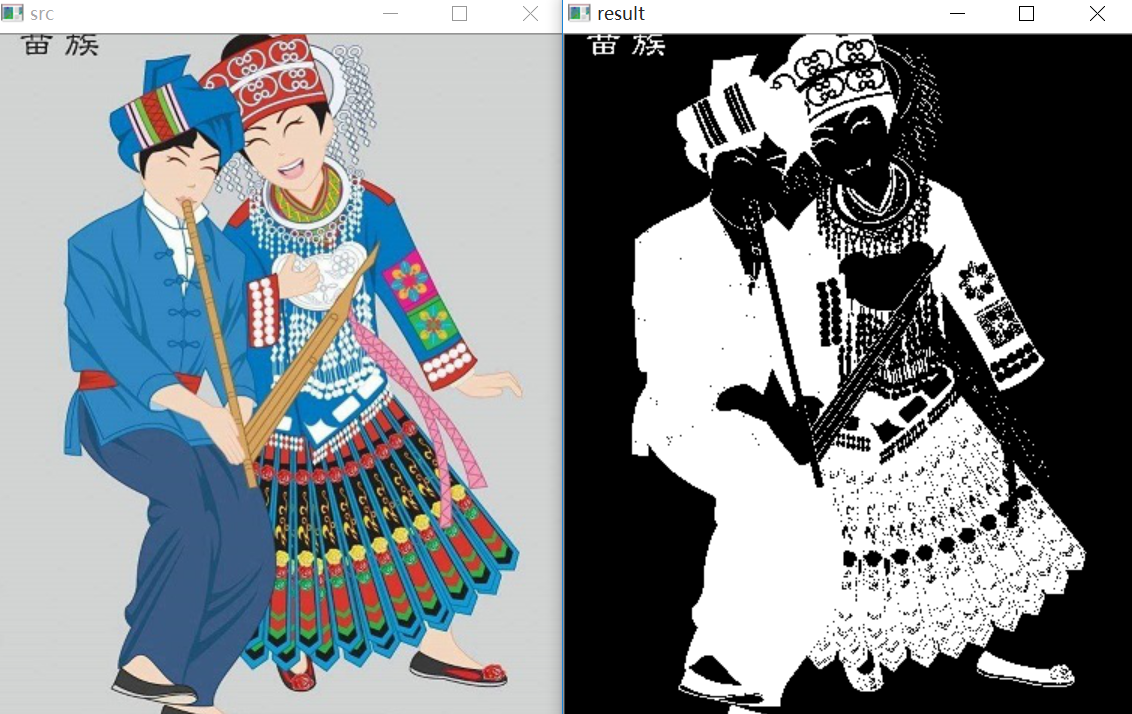# 四. 截断阈值化

$dst(x,y) = \begin{cases} threshold， if src(x,y)>thresh\\ src(x,y)，otherwise\\ \end{cases}$
(1) 大于等于127的像素点的灰度值设定为该阈值127
(2) 小于该阈值的灰度值不改变

#encoding:utf-8
import cv2
import numpy as np

#读取图片

#灰度图像处理
GrayImage = cv2.cvtColor(src,cv2.COLOR_BGR2GRAY)

#截断阈值化处理
r, b = cv2.threshold(GrayImage, 127, 255, cv2.THRESH_TRUNC)
print r

#显示图像
cv2.imshow("src", src)
cv2.imshow("result", b)

#等待显示
cv2.waitKey(0)
cv2.destroyAllWindows()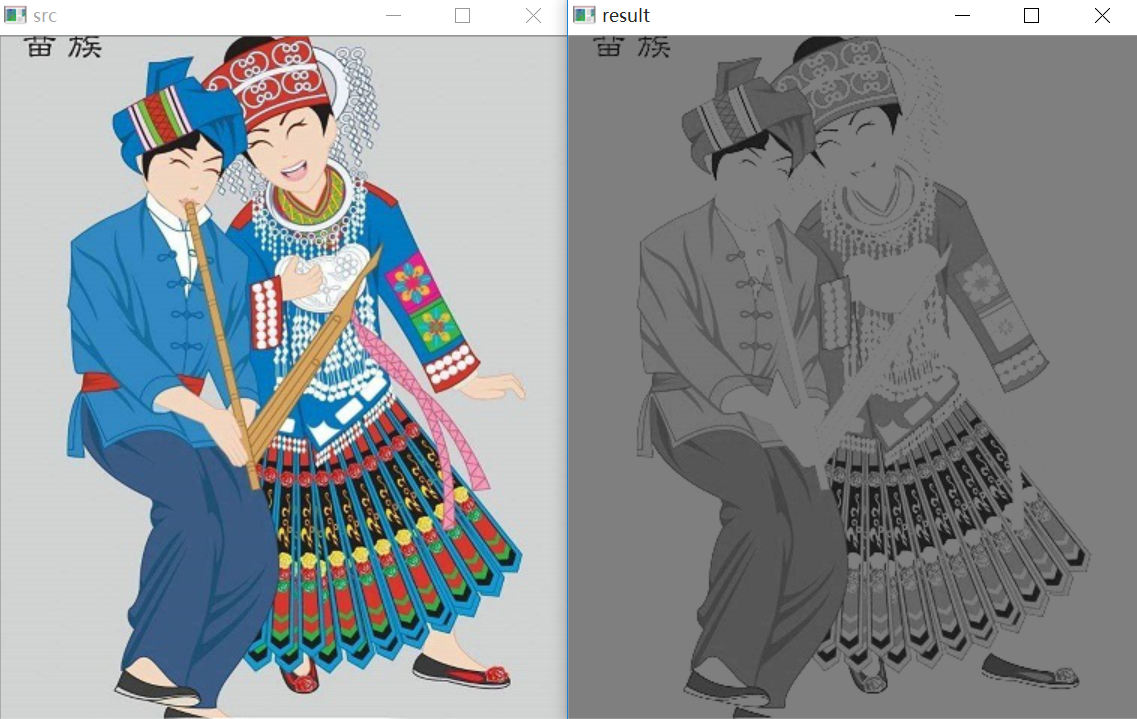# 五. 反阈值化为0

$dst(x,y) = \begin{cases} 0， if src(x,y)>thresh\\ src(x,y)，otherwise\\ \end{cases}$
(1) 大于等于阈值127的像素点变为0
(2) 小于该阈值的像素点值保持不变

#encoding:utf-8
import cv2
import numpy as np

#读取图片

#灰度图像处理
GrayImage = cv2.cvtColor(src,cv2.COLOR_BGR2GRAY)

#反阈值化为0处理
r, b = cv2.threshold(GrayImage, 127, 255, cv2.THRESH_TOZERO_INV)
print r

#显示图像
cv2.imshow("src", src)
cv2.imshow("result", b)

#等待显示
cv2.waitKey(0)
cv2.destroyAllWindows()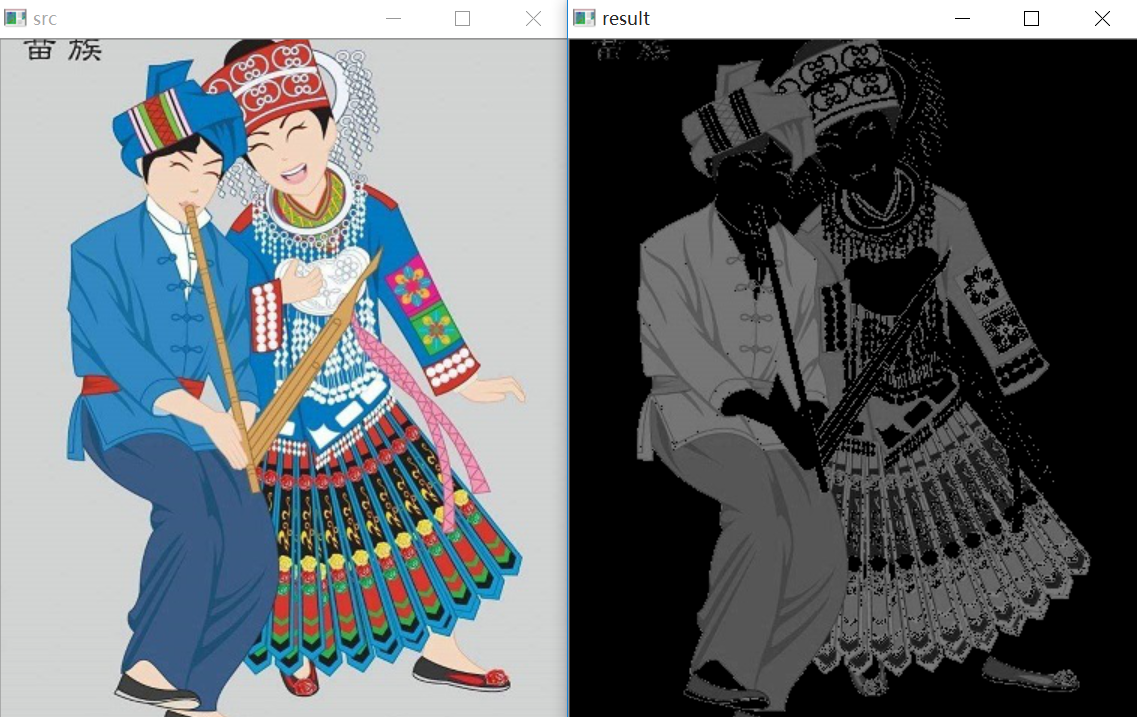# 六. 阈值化为0

$dst(x,y) = \begin{cases} src(x,y)， if src(x,y)>thresh\\ 0，otherwise\\ \end{cases}$
(1) 大于等于阈值127的像素点，值保持不变
(2) 小于该阈值的像素点值设置为0

#encoding:utf-8
import cv2
import numpy as np

#读取图片

#灰度图像处理
GrayImage = cv2.cvtColor(src,cv2.COLOR_BGR2GRAY)

#阈值化为0处理
r, b = cv2.threshold(GrayImage, 127, 255, cv2.THRESH_TOZERO)
print r

#显示图像
cv2.imshow("src", src)
cv2.imshow("result", b)

#等待显示
cv2.waitKey(0)
cv2.destroyAllWindows()#encoding:utf-8
import cv2
import numpy as np
import matplotlib.pyplot as plt

#读取图像
lenna_img = cv2.cvtColor(img,cv2.COLOR_BGR2RGB)
GrayImage=cv2.cvtColor(img,cv2.COLOR_BGR2GRAY)

#阈值化处理
ret,thresh1=cv2.threshold(GrayImage,127,255,cv2.THRESH_BINARY)
ret,thresh2=cv2.threshold(GrayImage,127,255,cv2.THRESH_BINARY_INV)
ret,thresh3=cv2.threshold(GrayImage,127,255,cv2.THRESH_TRUNC)
ret,thresh4=cv2.threshold(GrayImage,127,255,cv2.THRESH_TOZERO)
ret,thresh5=cv2.threshold(GrayImage,127,255,cv2.THRESH_TOZERO_INV)

#显示结果
titles = ['Gray Image','BINARY','BINARY_INV','TRUNC','TOZERO','TOZERO_INV']
images = [GrayImage, thresh1, thresh2, thresh3, thresh4, thresh5]
for i in xrange(6):
plt.subplot(2,3,i+1),plt.imshow(images[i],'gray')
plt.title(titles[i])
plt.xticks([]),plt.yticks([])
plt.show()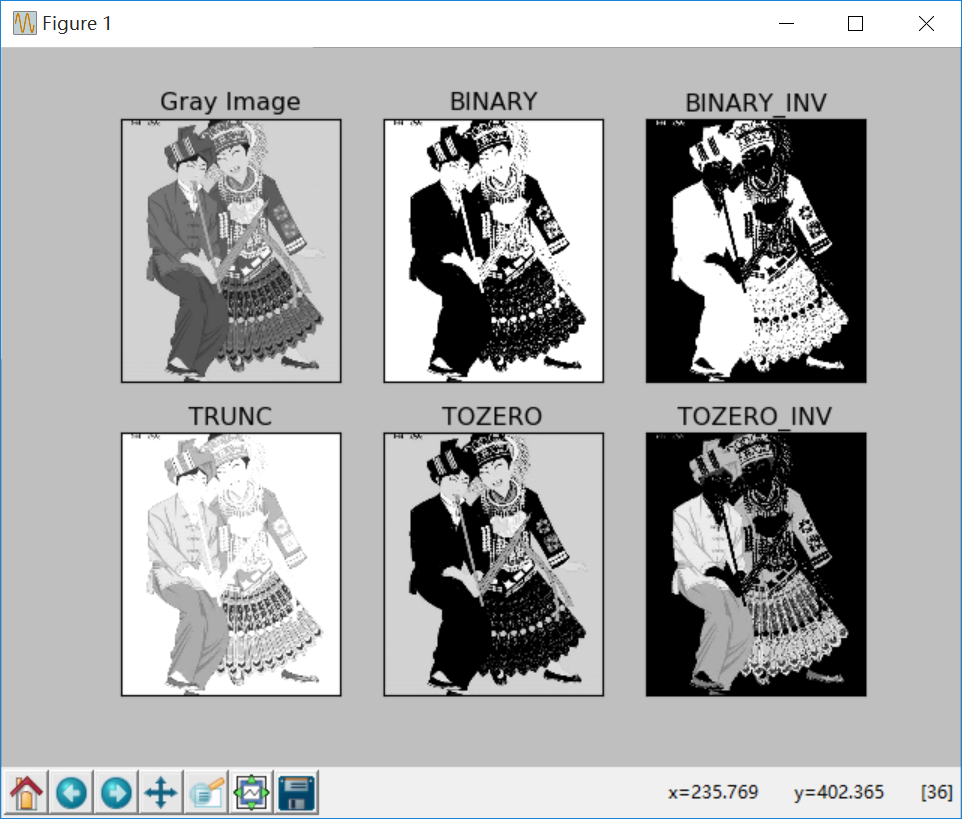（By：Eastmount 2018-10-30 晚上10点 https://blog.csdn.net/Eastmount/）

2013-12-04 18:43:27 xiaqunfeng123 阅读数 10258
• ###### Java经典算法讲解

在面试中，算法题目是必须的，通过算法能够看出一个程序员的编程思维，考察对复杂问题的设计与分析能力，对问题的严谨性都能够体现出来。一个算法的好坏，直接影响一个方法调用的性能，进而影响软件的整体性能。算法是学习所有编程语言的基础，在Java的学习过程中首先也会选择以算法起步，本次课程重点讲解Java开发中常用的基本算法。

29961 人正在学习 去看看 张中强

Otsu法是1979年由日本大津提出的。该方法在类间方差最大的情况下是最佳的，即统计鉴别分析中所用的度量。Otsu方法有一个重要的特性，就是它完全以在一幅图像的直方图上执行计算为基础，而直方图是很容易得到的一维阵列。

具体的公式推理及公式细节就不说了，详见 Conzalez 那本书，我是第三版的，在P.479——P.482 上面。

1、计算输入图像的直方图，并归一化。

2、计算累积均值mu，以及全局灰度均值。

3、计算被分到类1的概率q1，和被分到类2的概率q2。

4、用公式计算类间方差，sigma = q1*q2*(mu1 - mu2)*(mu1 - mu2)

5、循环寻找类间方差最大值，并记下此时的阈值，即为最佳阈值。

6、利用最佳阈值进行图像阈值化。

double getThreshVal_Otsu_8u( const Mat& _src )
{
Size size = _src.size();
const int N = 256;
int i, j, h[N] = {0};
unsigned char* src;
//直方图统计
for( i = 0; i < size.height; i++ )
{
src = _src.data + _src.step*i;
j = 0;
for(j = 0; j < size.width; j++ )
h[src[j]]++;
}
//像素平均值
double mu = 0, scale = 1./(size.width*size.height);
for( i = 0; i < N; i++ )
{
mu += i*(double)h[i];//累加均值
}
mu *= scale;//平均

double mu1 = 0, q1 = 0;//q1 ,q2 为类1和类2的概率累积和，mu1=mg*q1
double p_i, q2, mu2, sigma;
double max_sigma = 0, max_val = 0;
//循环求取最大阈值
for( i = 0; i < N; i++ )
{
p_i = h[i]*scale;//直方图归一化
mu1 *= q1;
q1 += p_i;
q2 = 1. - q1;
mu1 = (mu1 + i*p_i)/q1;
mu2 = (mu - q1*mu1)/q2;
sigma = q1*q2*(mu1 - mu2)*(mu1 - mu2);//类间方差
if( sigma > max_sigma )
{
max_sigma = sigma;
max_val = i;//记下使类间方差最大的阈值
}
}
return max_val;//返回阈值
}2010-05-26 21:36:00 yangfenghero 阅读数 1178
• ###### Java经典算法讲解

在面试中，算法题目是必须的，通过算法能够看出一个程序员的编程思维，考察对复杂问题的设计与分析能力，对问题的严谨性都能够体现出来。一个算法的好坏，直接影响一个方法调用的性能，进而影响软件的整体性能。算法是学习所有编程语言的基础，在Java的学习过程中首先也会选择以算法起步，本次课程重点讲解Java开发中常用的基本算法。

29961 人正在学习 去看看 张中强

阈值变换在图像处理中有着非常高的作用，很多处理都会用到。最常见的应用就是产生黑白图。

代码如下：